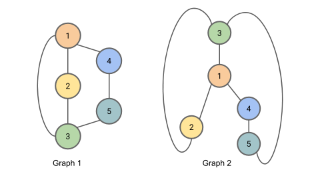# Graph isomorphism problem

Topic | v1 | created by janarez |
Description

The graph isomorphism problem is the computational problem of determining whether two finite graphs are isomorphic. The problem is not known to be solvable in polynomial time nor to be NP-complete, and therefore may be in the computational complexity class NP-intermediate. It is known that the graph isomorphism problem is in the low hierarchy of class NP, which implies that it is not NP-complete unless the polynomial time hierarchy collapses to its second level. At the same time, isomorphism for many special classes of graphs can be solved in polynomial time, and in practice graph isomorphism can often be solved efficiently.

##### Relations

Generalization of neural networks to arbitrary graphs.

##### Resources

7.0 rating 2.0 level 9.0 clarity 3.0 background – 1 rating

Two graphs are considered isomorphic if there is a mapping between the nodes of the graphs that prese...